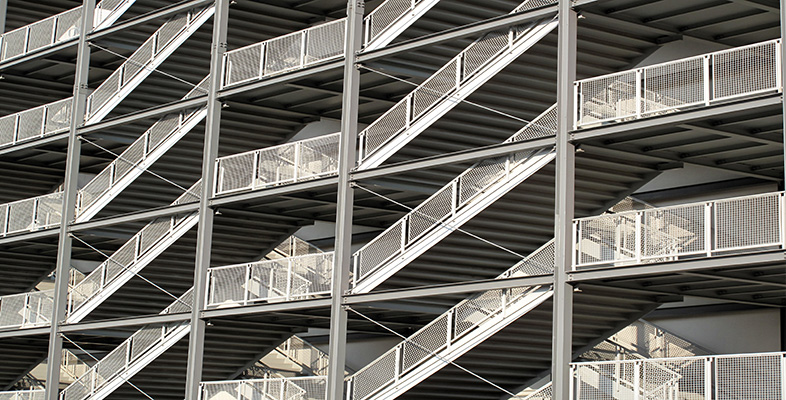Structural devices

This free course is available to start right now. Review the full course description and key learning outcomes and create an account and enrol if you want a free statement of participation.

Free course

4.3 PZT

The above requirements lead us to a range of ceramic materials with crystalline structure. One such material is lead zirconate titanate (PZT), which is an oxide alloy of lead, zirconium and titanium. It is often used in a specific composition (sometimes with additives) in order to achieve a particular crystal structure and the desired piezoelectric response. Figure 20 shows the structure of PZT.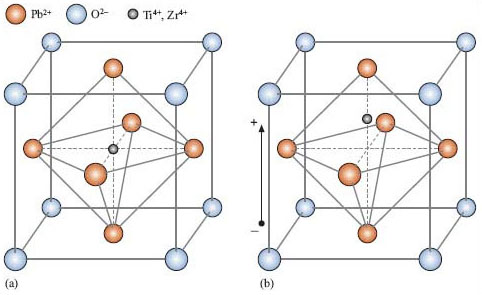Figure 20 (a) Structure of PZT; (b) under influence of an electric field

The choice of constituent atoms in the structure is no accident. The radii of the atoms and their bonding affinity lead to a lattice that is rigid, while affording some freedom to the positive ions, in the centre of the cages, to move within the structure.

To be useful as a piezoelectric material the PZT needs to be ‘poled’. This is done by heating the material within a large electric field (>2000 V mm−1). The material expands slightly along the axis of the electric field and contracts a little in the perpendicular direction. Upon cooling with the electric field still on, this distortion of the material is frozen in, resulting in a permanent polarisation even after the field is turned off. The PZT is now a ferroelectric material, in a technically useful state that is polarised in zero electric field. A field can now be applied over quite a range, which will strain the material causing a slight shape change. However, care must be taken not to exceed a field strength that would ‘depole’ the piece. In the other mode of operation, an applied stress causes a mechanical strain that, through displacing the ions in their cages, alters the polarisation, giving rise to a voltage across the material.

The most important quantity for describing the extent to which a material is piezoelectric is its coupling coefficient k. This expresses the efficiency of energy conversion between mechanical and electrical forms. It is defined by: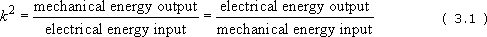which, for a poled ceramic, is: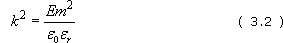where E is the Young's modulus of the material; m is the piezoelectric ‘motor’ coefficient, defined as the rate of change of strain with field; ε0 is the permittivity of free space (which approximately equals 8.85 × 1012 F m −1); and εr is the relative permittivity of the material.

SAQ 7

A particular PZT piezoelectric actuator has a coupling coefficient of 0.7. Determine how much more efficient this is in conversion of electrical to mechanical energy than quartz, which has a coupling coefficient of 0.1.

Energy interconversion is proportional to the square of the coupling coefficient. The ratio of energy conversion will be 0.72/0.12 = 49 times more efficient.

Figure 21 shows how the substitution of zirconium atoms for some of the titanium atoms in the structure can be used to control the coupling coefficient.

In summary, this has been a brief skirmish with the piezoelectric effect, the material requirements and the effect's atomic origins. PZT and its various compositions are some of many materials technically suited to use in piezoelectric applications, but are by no means the only ones.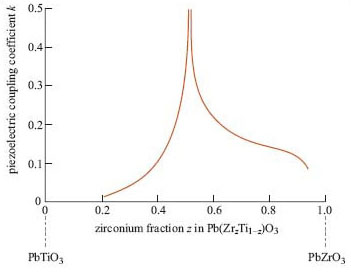Figure 21 Coupling coefficient versus composition for PZT
T356_1